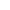CONVERTIONS SIMPLE MATH CONVERTIONS METRIC WEIGHTS AND MEASURES U.S. WEIGHTS AND MEASURES 1 CENTIMETER(cm)1 DECIMETER (dm)1 METER (m)1 DEKAMETER(dam)1 HECTOMETER(hm)1 KILOMETER (km)1 CENTILITER(cL)1 DECILITER(dL1 LITER(L)1 DEKALITER(daL)1 HECTOLITER(hL)1 KILOLETER(kL)1 CENTIGRAM(cg)1 DECIGRAM(dg)1 GRAM (g)1 DEKAGRAM(dag)1 HECTOGRAM(hg)1 KILOGRAM (kg)1 TON (t) 10 millimeters (mm)10 centimeters (cm)10 decimeters (dm)10 meters (m)10 dekameters (dam)10 hectometers (hm)10 mililiters (mL)10 centiliters (cL)10 deciliters (dL)10 liters (L)10 dekaliters (daL)10 hectoliters (hL)10 miligrams (mg)10 centigrams (cg)10 decigrams (dg)10 grams (g)10 dekagrams (dag)10 hectograms (hg)1,000 kilograms (kg) 12 inches (in)3 feet (ft)5,280 feet (ft)4 gills (gi)2 pints (pt)4 quarts (gt)27 11/32 grains16 drams (dr)16 ounces (oz)100 pounds (lbs)20 hundredweights (20,000 lbs) 1 FOOT (ft)1 YARD(yr)1 MILE (mi)1 PINT (pt)1 QUART (qt)1 GALLON (gal)1 DRAM (dr)1 OUNCE (oz)1 POUND(lb)1 HUNDREDWEIGHT (cwt)1 TON (t) LETS WORK THIS OUT. LETS SOLVE THE PROBLEM. You are going to the store and you are going to buy a kilo of table tortillas.  (the thicker kind of corn tortillas)  Each package has 40 corn tortillas, you want to know how many each tortilla weights.  (IN THIS EXCERCISE  KILO IS SHORT FOR KILOGRAM COMMON USED NAME.) WHAT WE KNOW: 1 kilo = 10 hg 1 hg = 10 dag 1 dag = 10 gSo lets find a way to compare.now we want to know how many grams are in a kilogram so we can compare the measurements by division. if 10 hgs are 1 kg then we sould set up as 10 hg                                                                                                            1 kgand one hectogram are 10 dekagrams so then we would write is as     10 dag                                                                                                             1 hgand since we know that 10 grams are 1 dekagram then it would be as 10 g                                                                                                           1 dagso we can have grams as a result . now lets multiply to solve . 1 kilo per pack( pak)     1 kg *   10 hg *   10 dag   *   10 g      = 1000 g     1 pak  *    1 kg    *    1 hg     *   1 dag    =  1 pak so every pack has 1000 grams ok now we know that eack pack has 40 tortillas (tr) so we write it we want to know how many each tortilla weights. so we do a conversion again.     1000 g = 1 pak   1 pack = 40 tortillas so we want to know grams per tortilla    g / tr   so we solve we want grams on top and tortillas on bottom, so we put    1 pak   *   1000 g    =  1000 g   so now we devide 1000 by 40 1000/40 = 25 g    40 tr    *    1 pak     =  40 trso each tortilla weighs 25 grams. HOME - ALGEBRA - GEOMETRY - CONVERTIONS THE TRICK HERE IS TO IS TO ALWAYS ALIGN THE EQUIVILANTS AS FRACTIONS, THEN SIMPLIFY. REMEMBER TO ALWAYS LEAVE WHAT YOU WANT TO FIND AS IN THIS PAST PROELM WE WANTED TO FIND GRAMS, SO WE PLACED IT ON THE TOP, THEN FOLLOW THE SEQUANCE. REMEMBER ALWAYS MULTIPLY AND AT THE END SIMPLIFY TO FIND THE ANSWER. IF YOU STILL DON'T GET IT EMAIL ME math_can_help@yahoo.com                                                                         or text me if I'm on line